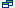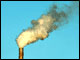Languages:#### Mass (weight) Units

The Metric System of Measurements uses the mass units: gram (g), kilogram (kg) and tonne (t).

 1000 g = 1 kg 1000 kg = 1 tonne Adding prefixes of the International System of Units (SI) allows to express weight as multiples or fractions of 1 gram: 1 tonne (t) =1 000 000 g 1 kilogram (kg) =1 000 g 1 gram (g) =1 g 1 milligram (mg) =0.001 g 1 microgram (µg) =0.000 001 g 1 nanogram (ng) =0.000 000 001 g 1 picogram (pg) =0.000 000 000 001g

Imperial and US weight units can also be expressed as metric units:

 1 US ton (ton) =0.907 tonne 1 UK ton (ton) =1.016 tonne 1 lb (pound) =453.59 g 1 oz (ounce) =28.35g

Further information on the International System of Units (SI) is provided by the International Bureau of Weights and Measures (BIPM) www.bipm.org/en/si/Related publication:Dioxins
Other Figures & Tables on this publication:

Table2. : Estimated tissue concentrations in human populations exposed to dioxin and dioxin-like compounds

Table 7: Estimated daily dietary intake of PCBs in the USA. a

JECFA ASSESSMENT

Dioxin Body Burden

Toxic Equivalents Scheme (TEFs & TEQs)

Daily Dioxin IntakesSome average daily intakes for long term exposures to dioxins:

Mass (weight) Units